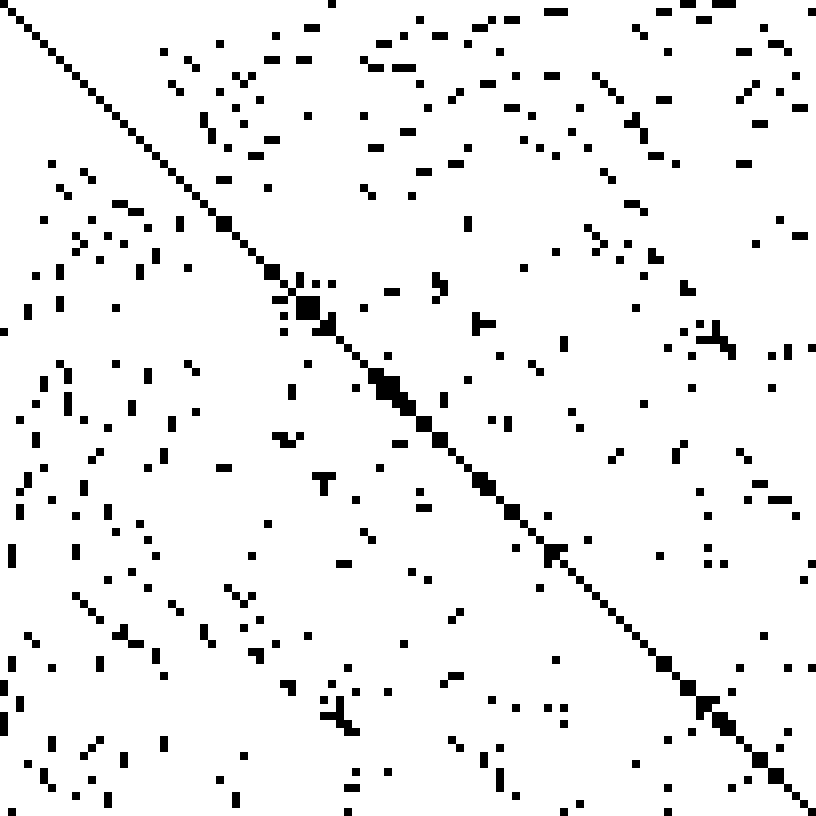# Code Aster solver: what are the differences?

During a FEM calculation it is important not only to be able to intelligently model a structure by making the necessary simplifications, but also, be able to understand the best solver is to use. The choice of the solver also influences the calculation times, improving or worsening them. CloudHPC is a service that is based on hours of use. For this reason is necessary optimizing the performance of the analysis. In this article we will therefore go to see which are the solvers in Code_Aster.

## SOLVER METHODS

The object to be solved is the sparse matrix. As shown in the figure 1, a sparse matrix is a matrix with zero as prevalent number.An example of a FEM Sparse Matrix. The black dots are the number while the white space are the zero.

To resolve it, two type of methods are avaible:

• The direct one, that solve the stiffness matrix K with a single method iteration using an internal algorithm to calculate the inverse matrix.
• The iterative one, that have a numerical approach. They use an iterative procedure to evaluate the matrix K.

The direct methods implemented as Code Aster solver are:

MULT_FRONT (avaible only in parallel Code_Aster version): robust solver, with to disadvise however for modelings requiring of the swivelling (mixed, incompressible finite elements), for the matrices generalized with connections as on the large models finite elements [R6.02.02]

LDLT: Universal solver but only on small size finite elements (<10^5 dofs). Beyond that, the method is very slow. With to disadvise for modelings of the swivelling (mixed finite elements, incompressible,…)

MUMPS: Universal performing and robust solver. With to use on all types of problems and in particular the large models finite elements (>10^6 dofs), even the very large models ( >10^7 dofs). Into nonlinear, it is often preferable to use MUMPS as a preconditioner, via the option PETSC+PRE_COND = ‘LDLT_SP’/’LDLT_DP’.

The iterative methods implemented are instead:

GCPC: Real symmetrical problem except those requiring obligatorily a detection of singularity (modal calculation). Into nonlinear, if the problem is real non symmetrical, one can use this provided solver that the matrix was made symmetrical.

PETSC (avaible only in parallel Code_Aster version): All types of problems real except those requiring obligatorly a detection of singularitu (modal calculation). With to use in priority on the nonlinear problems (with PRE_COND = ‘ LDLT_SP’) or on the problems “borders” (> 10^7 dofs).

In addition to the brief explanation of their main features, in the Code_Aster document U4.50.01 you will find all the various parameters to optimize the

Currently, only the serialized version of Code_Aster is implemented in CloudHPC and the MULT_FRONT and PETSC algorithm are not avaible.

## How to set the Code Aster solver

To set the solver method, go in analysis command, select the analysis you want (as example in this case a non linear solver has been selected) and go to the SOLVEUR command.

Click EDIT and a new tab will be displayed.

On METHODE select the method desired.

Depending on the type of analysis, a different algorythm will be necessary. The test has performed on MECA_STATIQUE and NON_LINE_MECA solver.

## Linear Analysis

With regard to linear static analysis (MECA_STATIQUE), the best algorithm you can use, both for strength and speed, is MUMPS. For more information on how to best set the parameters for your analysis, see the Code_Aster document.

## Code Aster solver for Non Linear Analysis

With regard to non linear static analysis (NON_LINE), a simple solid cyilinder is here analyzed. The material is a classic steel which has a geometric non-linearity (large deformations) despite having a linear elastic behavior (we are in any case below the yield threshold).

The boundary condition are:

1. Fixed on one face.

The model analyzed have the following mesh density:

1. 20 mm element dimension -> 20555 nodes -> 61665 dofs

The Code Aster solver taken in consideration are the following:

1. MUMPS;
2. LDLT;
3. GCPC;

For each methods has used the default features chosen by the software.

In the graph below are reporting the time rapported to the minimum value.